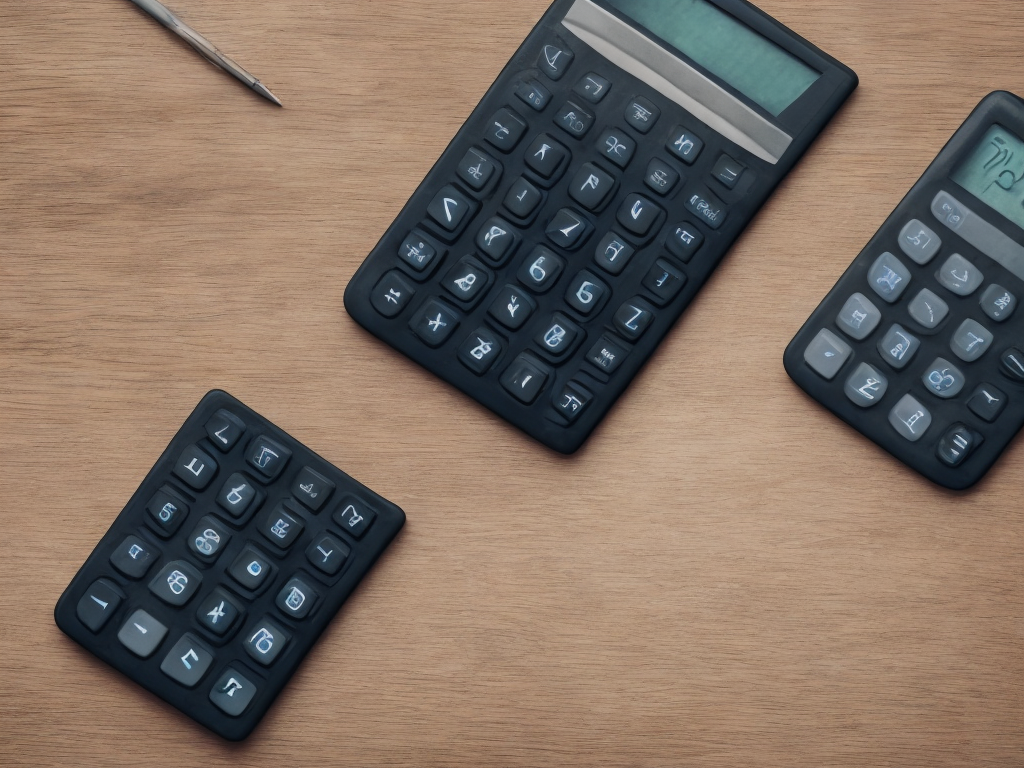# How To Find Percentage

tl;dr
To find a percentage, multiply the number by the percentage fraction (e.g. 20% of 100 = 100 x 20/100 = 20).Finding percentages is an essential math skill that is necessary in a wide range of applications. Whether you work in finance, engineering, or science, percentages play a critical role in data analysis. Plus, percentages are also an essential ingredient in everyday life, whether it's calculating discounts, taxes, or tips. In this article, we'll guide you through the process of finding percentages, step-by-step.

Percentage Basics

Before we dive into the techniques for finding percentages, let's review what percentages are in the first place. A percentage is a way of expressing a number as a portion or fraction of 100. For example, if we say that a certain investment has a return of 7%, we mean that it returns seven cents for every dollar invested, or a total of \$0.07 for every \$1. If we say that a sales tax rate is 8%, that means for every \$100 spent on taxable goods, the buyer must pay \$8 in taxes.

The formula for calculating percentages is straightforward. To find the percentage of a number, you take that number and multiply it by the percentage fraction. For instance, to find 20% of 100, you would multiply 100 by 20/100 or 0.2, resulting in an answer of 20. Similarly, to calculate 35% of 500, you would multiply 500 by 35/100, getting an answer of 175.

Now that we've covered some of the basics, let's explore some different methods for finding percentages.

Method #1: Percentage Calculator

One of the simplest ways to find a percentage is to use an online percentage calculator. There are dozens of calculators available online, and most of them work the same way. Simply enter the numbers you wish to calculate, click on the "calculate" button, and the percentage will be shown on the screen.

For instance, let's say you want to find out what 15% of 450 is. You can type "15%" and "450" into the calculator, and the result would be shown as 67.5.

Method #2: Percentage of a Whole Number

Calculating the percentage of a whole number involves finding out what percentage of the total that number represents. For example, if there are 40 apples in a basket, and 10 of them are rotten, we can say that 25% of the apples are ruined.

To calculate the percentage of a whole number, follow these steps:

1. Divide the part by the whole. In the example above, we would divide 10 by 40, resulting in 0.25.

2. Multiply the answer by 100 to convert it to a percentage. In our example, 0.25 multiplied by 100 results in 25%.

Method #3: Percent Increase or Decrease

Sometimes you need to calculate the percentage change in a value between two different periods. For instance, if a company's sales increased from \$10,000 in 2020 to \$12,000 in 2021, we can calculate the percentage increase by following these steps:

1. Subtract the old value from the new value. In our example, the new value of \$12,000 minus the old value of \$10,000 results in an increase of \$2,000.

2. Divide the increase by the old value. In this case, \$2,000 divided by \$10,000 results in 0.2 or 20%.

3. Multiply the answer by 100 to convert to a percentage. Therefore, the percentage increase is 20%.

Similarly, if the company's sales had decreased from \$12,000 in 2021 to \$9,000 in 2022, we could calculate the percentage decrease as follows:

1. Subtract the new value from the old value. In our example, the old value of \$12,000 minus the new value of \$9,000 results in a decrease of \$3,000.

2. Divide the decrease by the old value. In this case, \$3,000 divided by \$12,000 results in 0.25 or 25%.

3. Multiply the answer by 100 to convert to a percentage. Thus, the percentage decrease would be 25%.

Method #4: Percentage Conversion

Converting decimals or fractions to percentages is a simple process. To convert a fraction to a percentage, simply multiply the fraction by 100. For example, 0.5 is the same as 50%. To convert a decimal to a percentage, multiply the decimal by 100 and add the percent symbol. Hence, 0.25 is equal to 25%.

Method #5: Percentage as Proportions

Percentages can also be seen as proportions. To express a percentage as a proportion, we use fractions. For example, 25% can be written as 25/100, or simplified to 1/4. Similarly, 50% can be written as 50/100 or 1/2.

Conclusion

Calculating percentages may seem intimidating at first, but with a little practice, anyone can master the methods outlined in this article. Whether you're dealing with sales figures, budgeting, or everyday calculations, knowing how to find percentages is an essential skill that will serve you well in all aspects of life. With the right technique and a bit of practice, anyone can become a percentage pro, ready to tackle any calculation that comes their way.